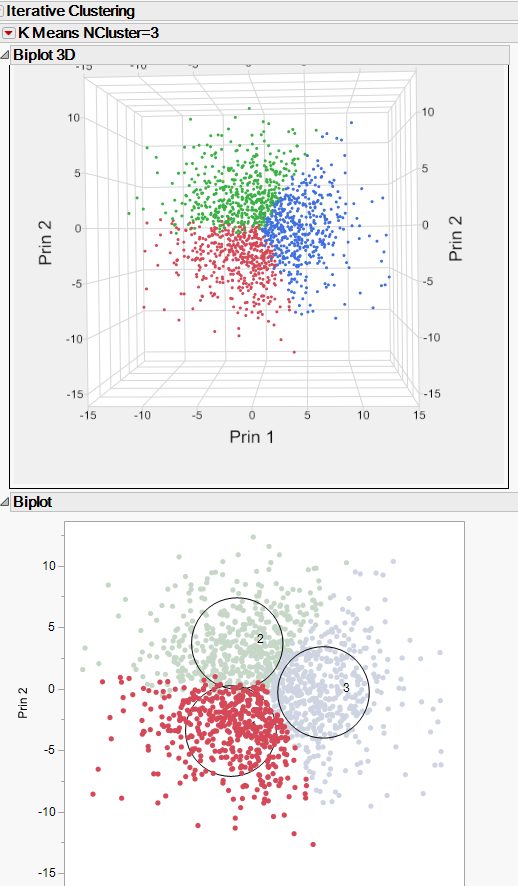Choose Language Hide Translation Bar
Highlighted

## What are the principal components in a 3D Biplot after k-means clustering?

Hi,

I have clustered a dataset with n=5008 using the kmeans clustering algorithm. One option for a visualization is the 3D biplot that uses 3 axes with the principal components. Unfortunately, I can not find a clear explanation where it comes from. My guess is, that simply the variables used for clustering are used for a principal component analysis, but i have no proof.

Seb

1 ACCEPTED SOLUTION

Accepted Solutions
Highlighted

## Re: What are the principal components in a 3D Biplot after k-means clustering?

I selected the "?" tool and clicked on the 3D biplot and the help screen for the K Means Clustering Report Options was displayed.  Here is part of its verbage:

Biplot
Shows a plot of the points and clusters in the first two principal components of the data. Circles are drawn around the cluster centers. The size of the circles is proportional to the count inside the cluster. The shaded area is the 50% density contour around the mean, and indicates where 50% of the observations in that cluster would fall (Mardia et al., 1980). Below the plot is an option to save the cluster colors to the data table. The eigenvalues are shown in decreasing order.
Biplot Options
Contains the following options for controlling the appearance of the Biplot:
Show Biplot Rays
Shows the biplot rays. The labeled rays show the directions of the covariates in the subspace defined by the principal components. They represent the degree of association of each variable with each principal component.
Biplot Ray Position
Enables you to specify the position and radius scaling of the biplot rays. By default, the rays emanate from the point (0,0). In the plot, you can drag the rays or use this option to specify coordinates. You can also adjust the scaling of the rays to make them more visible with the radius scaling option.
Mark Clusters
Assigns markers that identify the clusters to the rows of the data table.
Biplot 3D
Shows a three-dimensional biplot of the data. Available only when there are three or more variables.

And as seen below the 3D Biplot is just an expansion on the 2D Biplot, so the 2D Biplot definition also describes the 3D BiplotJim
2 REPLIES 2
Highlighted

## Re: What are the principal components in a 3D Biplot after k-means clustering?

I selected the "?" tool and clicked on the 3D biplot and the help screen for the K Means Clustering Report Options was displayed.  Here is part of its verbage:

Biplot
Shows a plot of the points and clusters in the first two principal components of the data. Circles are drawn around the cluster centers. The size of the circles is proportional to the count inside the cluster. The shaded area is the 50% density contour around the mean, and indicates where 50% of the observations in that cluster would fall (Mardia et al., 1980). Below the plot is an option to save the cluster colors to the data table. The eigenvalues are shown in decreasing order.
Biplot Options
Contains the following options for controlling the appearance of the Biplot:
Show Biplot Rays
Shows the biplot rays. The labeled rays show the directions of the covariates in the subspace defined by the principal components. They represent the degree of association of each variable with each principal component.
Biplot Ray Position
Enables you to specify the position and radius scaling of the biplot rays. By default, the rays emanate from the point (0,0). In the plot, you can drag the rays or use this option to specify coordinates. You can also adjust the scaling of the rays to make them more visible with the radius scaling option.
Mark Clusters
Assigns markers that identify the clusters to the rows of the data table.
Biplot 3D
Shows a three-dimensional biplot of the data. Available only when there are three or more variables.

And as seen below the 3D Biplot is just an expansion on the 2D Biplot, so the 2D Biplot definition also describes the 3D BiplotJim
Highlighted

## Re: What are the principal components in a 3D Biplot after k-means clustering?

Hi Jim,

thank you for your answer. I think I am on the right track. I'm still wondering about the definition. It says the "principal components of the data". It is not clear if the PCA ist based on the whole dataset and used in the visualization of the kmeans-clustering result or it is based in the variables used for clustering. I guess it is the latter case, It is not clearly stated though.

Thanks,

Seb

Article Labels

There are no labels assigned to this post.